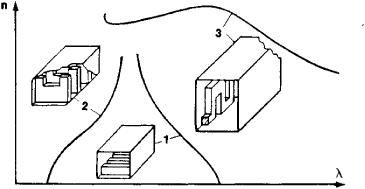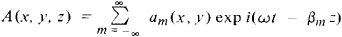# Periodic Structure

The following article is from The Great Soviet Encyclopedia (1979). It might be outdated or ideologically biased.

## Periodic Structure

in microwave technology, a structure, or system, that can be superimposed on itself by a parallel displacement over a certain finite distance. The minimum value d of this distance is called the period. Strictly speaking, periodic structures are infinite and serve as idealized models for the theoretical study of real objects. In practice, limited portions of periodic structures are used and are by convention also called periodic structures. Depending on the number of independent directions of displacement, we speak of one-dimensional, two-dimensional, or three-dimensional periodic structures (Figures 1 and 2). One-dimensional and two-dimensional periodic structures are employed as slow-wave systems, antennas, and diffraction gratings. Two-dimensional and three-dimensional periodic structures are used to construct lenses, prisms, and other devices that fix the direction of propagation of electromagnetic waves.Figure 1. One-dimensional periodic structures with various types of dispersion characteristics: (1) ridged wave guide having normal positive dispersion, (2) diaphragmed rectangular wave guide having negative dispersion, (3) meander wave guide having portions with anomalous and normal positive dispersion, (n) retardation coefficient, (λ) wavelength

Any component A of the electric and magnetic fields at a point of a periodic structure with the coordinate z (the direction of the structure’s periodicity coincides with the z-axis) can be represented as a series:Each term of the series is called a space harmonic. Here, am is the amplitude of the mth space harmonic and depends on the form of the periodic structure, ω is the angular frequency of the electromagnetic oscillations, t is the time, βm = β + (2πm/d) is the wave number of the mth space harmonic, and i is the unit imaginary number. The basic characteristics of a periodic structure are (1) the space harmonics’ retardation coefficients nm = βmc/ω, which by definition agree with the refractive index in optics and are numerically equal to the ratios of the phase velocity c of the wave in free space to the phase velocities ω/βm of the harmonics in the periodic structure; (2) the group velocity /m, which coincides in direction with the direction of energy transfer for the electromagnetic waves; and (3) the dispersion, which characterizes the dependence of the retardation coefficient n on the wavelength λ in free space. The phase velocity of the wave is determined from the retardation coefficient, and the group velocity can be obtained from the dispersion. The phase velocities and retardation coefficients of the space harmonics differ, but the harmonics’ group velocities are identical.

In microwave electronic devices that use periodic structures as interaction structures, the velocity of the electrons is usuallyFigure 2. (a) Two-dimensional periodic structure, (b) three-dimensional periodic structure: (d1), (d2), (d3) periods of the structures

close to the phase velocity of the wave but may differ from the group velocity in both magnitude and direction. Agreement between the directions of the phase and group velocities of a wave (positive dispersion) is typical for an oscillation-amplifying mode, and opposing directions for these velocities (negative dispersion) is characteristic for an oscillation-generating mode.

### REFERENCES

Aizenberg, G. Z. Antenny ul’trakorotkikh voln. Moscow, 1957.
Taranenko, Z. I., and Ia. K. Trokhimenko. Zamedliaiushchie sistemy. Kiev, 1965.
Silin, R. A., and V. P. Sazonov. Zamedliaiushchie sistemy. Moscow, 1966.

R. A. SILIN

References in periodicals archive ?
Inside every lithium-ion battery are particles whose atoms are arranged in a lattice-a periodic structure with gaps between the atoms.
A., "Periodic structure and photonic band-gap terminology: Historical perspectives," IEEE 29th European Microwave Conference, Vol.
Furthermore, the scattering of a large-scale periodic structure consisting of [N.sub.0] = 32 x 32 = 1024 element cells is considered.
Because the tuned blisk is a typical cyclic periodic structure, the modal coordinates of the boundary DOFs can be partitioned into [p.sub.b] = [[[p.sup.s.sub.b,r], [p.sup.s.sub.b,l].sup.T], and the following relationship can be obtained:
This way, we get the vibration transmission characteristics of this finite periodic structure to analyze the bandgap and vibration transmission pattern of this structure.
D-spacing length (DSL) was measured with the section profile in Figure 1(c) due to the periodic structure of collagen fiber.
The photonic band gap range for transverse electric (TE) modes for the periodic structure obtained by the PWE method is 0.24381 to 0.41527 as shown in Fig.
Each single harmonic does not satisfy the boundary conditions of the periodic structure, but their summation does so.
1D multiple quantum well PCs with a periodic structure of [(AB).sup.N] were considered as shown in Figure 1, where A and B represent PC consisting of two different dielectric materials with the refractive indices of [n.sub.A], [n.sub.B] and thicknesses of [d.sub.A] and [d.sub.B].
To the best of our knowledge, the 90 nm wide, 60 nm high periodic structure is one of the highest resolution sol-gel silica thin film structures ever fabricated.
To the STL problem of the periodic sandwich structure as stated above, the geometrical and characteristic parameters have a direct influence on the sound insulation performance, and the STL performance of periodic structure can be characterized by one periodic element.

Site: Follow: Share:
Open / Close